# How to remove text before the first match of a specific character

This post explains that how to remove text before the first occurrence of the comma character in a text string in excel. How to remove all characters before the first match of the comma or others specific characters in excel.

## Remove text before the first match of a specific character

If you want to remove all characters that before the first occurrence of the comma character, you can use a formula based on the RIGHT function, the LEN function and the FIND function.

You can use the FIND function to get the position of the first match of the comma character in a text string in Cell B1, then using the length value of text subtract the position value returned by the FIND function to get the length of substring that after the first comma.

Next, you can use the RIGHT function to extract the right-most characters after the first comma. So you can write down the following formula:

`=RIGHT(B1,LEN(B1)-FIND(",",B1))`

Let’s see how this formula works:

=FIND(“,”,B1)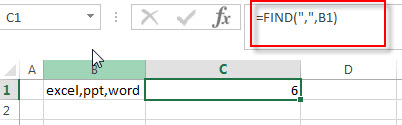This formula returns the position of the first occurrence of the comma character in a text string in Cell B1, it returns 6

=LEN(B1)-FIND(“,”,B1)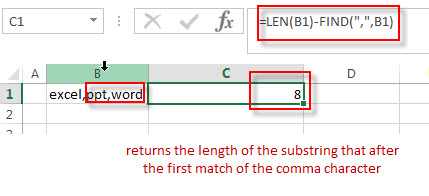This formula returns the length of the substring that after the first match of the comma character in a text string in Cell B1. It returns 14, and then it goes into the RIGTH function as its num_chars argument.

=RIGHT(B1,LEN(B1)-FIND(“,”,B1))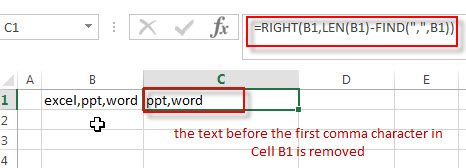This formula will extract the right-most 14 characters in a text string in Cell B1, so you can see that the text before the first comma character in a text string is removed.

If you want to remove characters before the first occurrence of the space character or others specific characters, you can replace the comma with space or others that you need.

`=RIGHT(B1,LEN(B1)-FIND(" ",B1))`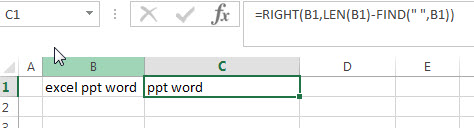## Remove text using Find &Select command

You can also use the Find and Replace command to remove text before a specified character, just refer to the following steps:

1# Click “HOME“->”Find&Select”->”Replace…”, then the window of the Find and Replace will appear.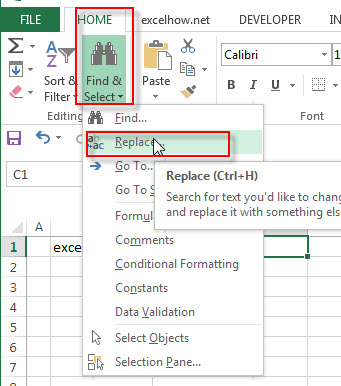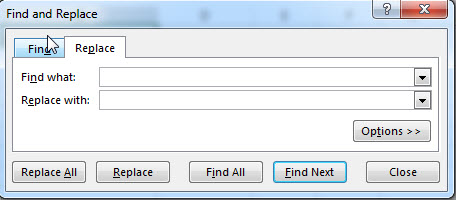2# click “Replace” Tab, then type *, into the Find what: text box, and leave blank in the Replace with: text box.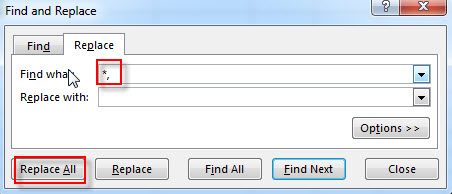3# click “Replace All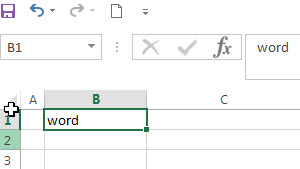You will see that all characters before the first comma character are removed.

### Related Functions

• Excel LEN function
The Excel LEN function returns the length of a text string (the number of characters in a text string).The LEN function is a build-in function in Microsoft Excel and it is categorized as a Text Function.The syntax of the LEN function is as below:= LEN(text)…
• Excel FIND function
The Excel FIND function returns the position of the first text string (sub string) within another text string.The syntax of the FIND function is as below:= FIND(find_text, within_text,[start_num])…
• Excel RIGHT function
The Excel RIGHT function returns a substring (a specified number of the characters) from a text string, starting from the rightmost character.The syntax of the RIGHT function is as below:= RIGHT (text,[num_chars])…